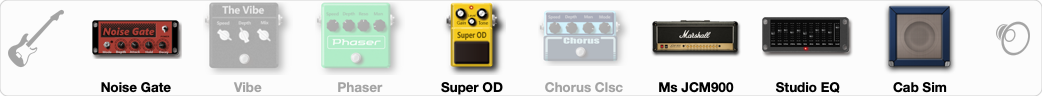# Zakk Wylde

Discussion in 'ToneLib-GFX presets' started by Jozef Stiavnicky, Jul 12, 2019.

1. ### Jozef StiavnickyNew Member

Zakk Wylde
Preset name: Zakk Wylde

Effects chain:Effect: "Noise Gate" (Dynamics / Filter), active - "yes"
"Detect" = Gtr In
"Mode" = Manual
"Depth" = 30
"Threshold" = 78
"Attack" = 3
"Hold" = 5
"Decay" = 250

Effect: "Vibe" (Modulation / Sfx), active - "no"
"Speed" = 1.0
"Depth" = 58
"Mix" = 50

Effect: "Phaser" (Modulation / Sfx), active - "no"
"Model" = MXR Phase 90
"Speed" = 2.1
"Depth" = 100
"Reso" = 14
"Freq" = 5.0

Effect: "Super OD" (Overdrive / Distortion), active - "yes"
"Drive" = 66
"Tone" = 58
"Level" = 66

Effect: "Chorus Clsc" (Modulation / Sfx), active - "no"
"Speed" = 2.9
"Depth" = 70
"Center" = 1.0
"Mode" = Stereo

Effect: "Ms JCM900" (Amp simulators), active - "yes"
"Gain" = 90
"Bass" = 90
"Middle" = 20
"Treble" = 40
"Presence" = 30
"Master" = 66
"Output" = 50
"Level (dB)" = 0

Effect: "Studio EQ" (Dynamics / Filter), active - "yes"
"31 Hz" = -15
"62 Hz" = -6
"125 Hz" = -10
"250 Hz" = 4
"500 Hz" = 0
"1 kHz" = 3
"2 kHz" = -3
"4 kHz" = 3
"8 kHz" = 0
"16 kHz" = -3
"above 16 kHz" = 0
"Level (dB)" = 0

Effect: "Cab Sim" (Cabinets), active - "yes"
"Model" = 4x12" 1960 T75
"Level (dB)" = 0

Note: You will need to download and install the ToneLib-GFX software to use the preset.

#### Attached Files:

• ###### Zakk_Wylde.tlgfx
File size:
693 bytes
Views:
7,100
Last edited: Jul 12, 2019
ARSZ, Nolr, CowboyFromHell63 and 3 others like this.